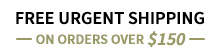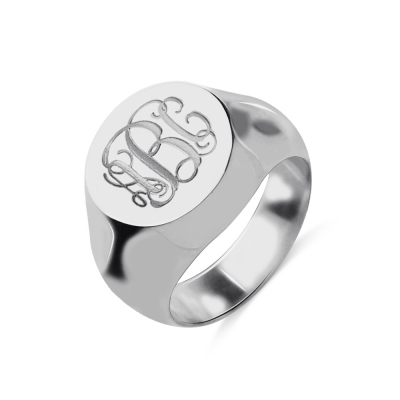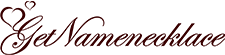## Roman numeral origin

Roman numerals, as the name suggests, are the number system used by the ancient Romans. In Roman numerals, there are 7 basic numbers, that is, I, V, X, L, C, D, and M, which represent 1, 5, 10, 50, 100, 500, and 1000, respectively. It can be noted that in this number system, 0 is not considered as an integer. It is said that, once a Roman scholar disregarded the Pope's opposition, he insisted on spreading the knowledge related to 0 and the role of 0 in the operation to the people. Therefore, he was imprisoned by the Pope and put into prison, on the grounds that 0 is an evil thing, destroying the Holy number.Similarly, Roman numerals cannot represent decimals (Note: Roman numerals have a fractional representation method, which can only represent integer multiples of 1/12). Therefore, Roman numerals are often used to indicate the year. In ancient books, architecture and clocks in European countries, we can all see the figures of Roman numerals. The familiar periodic table of elements also uses Roman numerals to indicate the "family" where the element is located. It should be noted that roman numerals are a counting rule, not a calculation rule. This means that roman numerals do not have the concept of carry and weight. Therefore, roman numerals are generally used for counting and not for calculation.

## Numerals conversion implementation- Roman Numeral Converter

After understanding the historical origins of Roman numerals, we have a certain understanding of Roman numerals. Now consider a problem, that is, the conversion between Roman numerals and Arabic numerals. Roman numerals were indeed invented by the ancient Romans, but Arabic numerals were actually invented by the ancient Indians. Today, people around the world use Arabic numerals, so a converter is needed between the two. This is exactly the problem we discussed at the beginning: If the people of the galaxy used Roman numerals to count, how would a rich man from earth trade with them when he arrived in the galaxy. Obviously, this conversion should be two-way, let's see how to achieve the corresponding conversion separately.

## Arabic numerals to Roman numerals

First, consider the conversion of Arabic numerals to Roman numerals, because a Roman numeral must be decremented from left to right, so we only need to arrange these 7 basic numbers from large to small and find the first numerical digit that is not less than the specified number. For example, 1024 obviously exceeds 1000, and 1000 in Roman numerals corresponds to M, so the first numerical digit of 1024 should be M. The next 24, obviously exceeds 10, so the second numerical digit of 1024 should be X. The next 14, obviously exceeds 10, so the third numerical digit of 1024 is also X. Next 4, this is a special number that needs to be represented as IV, which is the fourth numerical digit of 1024. If we connect the whole process in series, we can get the Roman numeral form MXXIV of 1024. One thing we noticed is that two numbers 4 and 9 are needed as auxiliary numbers here, because the numbers 1 to 3, 6 to 8 can always be obtained by repeatedly repeating 1, just like division.

GetNameNecklace.com: Your personalized jewelry online store.Welcome to Getnamenecklace

Our friendly support representatives will be around if you need any help

Please select the contact methods below

GET CONNECTED